## UGC NET CS 2017 Nov- paper-3

 Question 1
In 8085 microprocessor which of the following flag(s) is (are) affected by an arithmetic operation ?
 A AC flag Only B CY flag Only C Z flag Only D AC, CY, Z flags
Computer-Organization       Microprocessor
Question 1 Explanation:
AC Flag: This flag is set if thee is a carry to fifth bit from the fourth bits if two numbers.Question 2
In 8085 microprocessor the address bus is of __________ bits.
 A 4 B 8 C 16 D 32
Computer-Organization       Microprocessor
Question 2 Explanation:
In 8085 microprocessor the address bus is of 16 bits and data bus is 8 bits.
 Question 3
In the architecture of 8085 microprocessor match the following:A (a)-(iv), (b)-(i), (c)-(ii) B (a)-(iii), (b)-(iv), (c)-(ii) C (a)-(ii), (b)-(iii), (c)-(i) D (a)-(i), (b)-(ii), (c)-(iv)
Computer-Organization       Microprocessor
Question 3 Explanation:
Processing unit→ ALU
Arithmetic logic unit(ALU) is a fundamental building block of many types of computing circuits, including the central processing unit (CPU) of computers, FPUs, and graphics processing units (GPUs).
Instruction unit →Timing and control
Timing and Control unit is responsible for hardwired control unit and Microprogrammed control unit
Storage and Interface unit → General purpose Register
The general purpose register can store a data (or) a memory location address.
 Question 4
Which of the following addressing mode is best suited to access elements of an array of contiguous memory locations ?
Data-Structures       Arrays
Question 4 Explanation:
Index mode:
The address of the operand is obtained by adding to the contents of the general register (called index register) a constant value. The number of the index register and the constant value are included in the instruction code.
→ Index Mode is used to access an array whose elements are in successive memory locations (or) contiguous memory locations.
 Question 5
Which of the following is correct statement ?
 A In memory - mapped I/O, the CPU can manipulate I/O data residing in interface registers that are not used to manipulate memory words. B The isolated I/O method isolates memory and I/O addresses so that memory address range is not affected by interface address assignment. C In asynchronous serial transfer of data the two units share a common clock. D In synchronous serial transmission of data the two units have different clocks.
Computer-Organization       Isolated-vs-Memory-mapped-I/O
Question 5 Explanation:
FALSE: In memory - mapped I/O, the CPU can manipulate I/O data residing in interface registers that are used to manipulate memory words.
TRUE: The isolated I/O method isolates memory and I/O addresses so that memory address range is not affected by interface address assignment.
FALSE: In asynchronous serial transfer of data the two units share a different clock.
FALSE: In synchronous serial transmission of data the two units have common clocks.
 Question 6
A microinstruction format has micro-ops field which is divided into three subfields F1, F2, F3 each having seven distinct micro-operations, condition field CD for four status bits, branch field BR having four options used in conjunction with address field ADF. The address space is of 128 memory locations. The size of micro-instruction is:
 A 17 B 20 C 24 D 32
Computer-Organization       Microprogrammed-Control-Unit
Question 6 Explanation:
Given data, -- Micro-ops field F1 = 7 distinct micro operations
-- Micro-ops field F2 = 7 distinct micro operations
-- Micro-ops field F3 = 7 distinct micro operations
-- Condition field(CD) = 4 status bits
-- Branch Field(BR) = 4 options
-- Address space =128 memory locations
-- Size of micro operation=?
Given microinstruction formatQuestion 7
Consider the following four schedules due to three transactions (indicated by the subscript) using read and write on a data item X, denoted by r(X) and w(X) respectively. Which one of them is conflict serializable ?
S1: r1(X); r2(X); w1(X); r3(X); w2(X)
S2: r2(X); r1(X); w2(X); r3(X); w1(X)
S3: r3(X); r2(X); r1(X); w2(X); w1(X)
S4: r2(X); w2(X); r3(X); r1(X); w1(X)
 A S1 B S2 C S3 D S4
Database-Management-System       Transactions
Question 7 Explanation: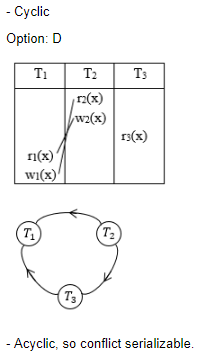Question 8
Suppose a database schedule S involves transactions T1, T2, .............,Tn. Consider the precedence graph of S with vertices representing the transactions and edges representing the conflicts. If S is serializable, which one of the following orderings of the vertices of the precedence graph is guaranteed to yield a serial schedule ?
 A Topological order B Depth - first order C Breadth - first order D Ascending order of transaction indices
Database-Management-System       Transactions
Question 8 Explanation:
If a schedule is conflict serializable then no cycle in precedence graph should be present.
But BFS and DFS are also possible for cyclic graphs.
And topological sort is not possible for cyclic graph.
Moreover option (D) is also wrong because in a transaction with more indices might come before lower one.
 Question 9
If every non-key attribute is functionally dependent on the primary key, then the relation is in __________ .
 A First normal form B Second normal form C Third normal form D Fourth normal form
Database-Management-System       Normalization
Question 9 Explanation:
1NF : A relation is in 1NF if it does not contain multi-value attributes and composite attributes.
2NF: A relation is in 2NF is there is no partial dependency exist in the relation. i.e.
primary_key → non-key attribute
prime_attribute → non-key attribute.
3NF : A relation is in 3NF if there is no transitive dependency or we can say that a relation is in 3NF if either LHS of a functional dependency is a super key or the RHS of the functional dependency is a prime key attribute.
4NF : A relation is in 4NF if there is no multivalued dependency exist in the relation.
Hence the correct answer is option (C)
NOTE: A prime key attribute is a attribute which is the part of primary key attributes. For example: If ABC is the the primary key then A,B,C, AB, AC, BC are the prime key attributes.
 Question 10
Consider a relation R (A, B, C, D, E, F, G, H), where each attribute is atomic, and following functional dependencies exist.
CH → G
A → BC
B → CFH
E → A
F → EG
The relation R is __________ .
 A in 1NF but not in 2NF B in 2NF but not in 3NF C in 3NF but not in BCNF D in BCNF
Database-Management-System       Normalization
Question 10 Explanation:
The attribute D is not part of any FD's. So, D can be a candidate key or it may be part of the candidate key.
Now D+ = {D}.
Hence we have to add A,B,C,E,F,G,H to D and check which of them are Candidate keys of size 2.
BD+ = {ABCDEFGH}
ED+ = {ABCDEFGH}
FD+= {ABCDEFGH}
But CD+, GD+ and HD+ does not give all the attributes hence CD, GD and HD are not candidate keys.
Here Candidate keys are AD, BD, ED and FD.
A → BC, B → CFH and F → EG etc are partial dependencies.
So given relation is in 1NF, but not in 2NF.
 Question 11
Given two relations R1(A, B) and R2(C, D), the result of following query Select distinct A, B from R1, R2 is guaranteed to be same as R1 provided one of the following condition is satisfied.
 A R1 has no duplicates and R2 is empty. B R1 has no duplicates and R2 is non - empty. C Both R1 and R2 have no duplicates. D R2 has no duplicates and R1 is non - empty.
Database-Management-System       SQL
Question 11 Explanation:
Option(A): Cartesian product with a empty table will result in zero tuple because we can't have any ordered pair with a empty table. Hence option(A) is incorrect option.
Option(B):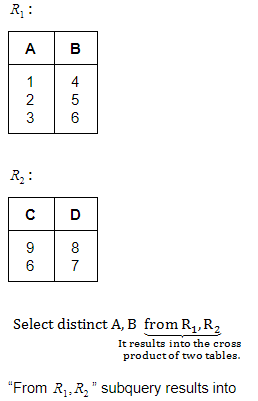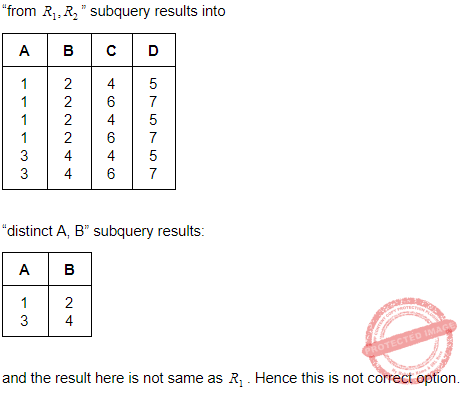Question 12
Consider a schema R(A, B, C, D) and following functional dependencies.
A → B
B → C
C → D
D → B
Then decomposition of R into R1(A, B), R2(B, C) and R3(B, D) is __________ .
 A Dependency preserving and lossless join. B Lossless join but not dependency preserving. C Dependency preserving but not lossless join. D Not dependency preserving and not lossless join.
Database-Management-System       Functional-Dependency
Question 12 Explanation:
(A, B) (B, C) - common attribute is (B) and due to B→C, B is a key for (B, C) and hence ABC can be losslessly decomposed into (A, B)and (B, C).
(A, B, C) (B, D) - common attributes is B and B→D is a FD (via B→C, C→D), and hence, B is a key for (B, D). So, decomposition of (A, B, C, D) into (A, B, C) (B, D) is lossless.
Thus the given decomposition is lossless.
The given decomposition is also dependency preserving as the dependencies A→B is present in (A, B), B→C is present in (B, C), D→B is present in (B, D) and C→D is indirectly present via C→B in (B, C) and B→D in (B, D).
 Question 13
Which of the following is not a component of Memory tube display ?
 A Flooding gun B Collector C Ground D Liquid Crystal
Computer-Graphics       Display-System
Question 13 Explanation:
Memory tube display components:
1. Connector Pins
2. Electron Gun
3. Base
4. Focusing System
5. Control Grid Voltage
6. X/Y Deflect
7. Phosphor
8. Collector
9. Ground
Note: Liquid crystal is not a component of memory tube display
.
 Question 14
Which of the following is not true in case of Oblique Projections?
 A Parallel projection rays are not perpendicular to the viewing plane. B Parallel lines in space appear parallel on the final projected image. C Used exclusively for pictorial purposes rather than formal working drawings. D Projectors are always perpendicular to the plane of projection.
Computer-Graphics       Oblique-Projections
 Question 15
With respect to CRT, the horizontal retrace is defined as:
 A The path an electron beam takes when returning to the left side of the CRT. B The path an electron beam takes when returning to the right side of the CRT. C The technique of turning the electron beam off while retracing. D The technique of turning the electron beam on/off while retracing.
Computer-Graphics       Display-System
 Question 16
Find the equation of the circle x2+y2=1 in terms of x'y' coordinates, assuming that the xy coordinate system results from a scaling of 3 units in the x' direction and 4 units in the y' direction.
 A 3(x')2 + 4(y')2 = 1 B (x'/3)2 + (y'/4)2 = 1 C (3x')2 + 4(y')2 = 1 D 1/3(x')2 + 1/4(y')2 = 1
Engineering-Mathematics       Co-ordinate-Geometry
 Question 17
Find the normalization transformation that maps a window whose lower left corner is at (1, 1) and upper right corner is at (3, 5) onto a viewport that is the entire normalized device screen.
 AB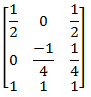CD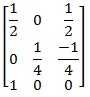Database-Management-System       Normalization
 Question 18
The three aspects of Quantization, programmers generally concerned with are:
 A Coding error, Sampling rate and Amplification B Sampling rate, Coding error and Conditioning C Sampling rate, Aperture time and Coding error D Aperture time, Coding error and Strobing
Computer-Networks       Quantization
 Question 19
The logic of pumping lemma is an example of __________.
 A iteration B recursion C the divide and conquer principle D the pigeonhole principle
Engineering-Mathematics       Combinatorics
Question 19 Explanation:
→ A pumping lemma (or) pumping argument states that, for a particular language to be a member of a language class, any sufficiently long string in the language contains a section, or sections, that can be removed, or repeated any number of times, with the resulting string remaining in that language.
→ The proofs of these lemmas typically require counting arguments such as the pigeonhole principle.
→ Hence, the logic of pumping lemma is a good example of the pigeonhole principle.
 Question 20
Heap allocation is required for languages that
 A use dynamic scope rules B support dynamic data structures C support recursion D support recursion and dynamic data structures
Compiler-Design       Run-Time-Environment
Question 20 Explanation:
→ Heap allocation is required for languages that support dynamic data structures. The heap is managed via calls to new, delete, callac, realloc, malloc, free, etc.
→ Stack allocation is required for local variables. Space on the stack is reserved for local variables when they are declared.
 Question 21
Pumping lemma for regular language is generally used for proving:
 A whether two given regular expressions are equivalent B a given grammar is ambiguous C a given grammar is regular D a given grammar is not regular
Theory-of-Computation       Regular-Language
Question 21 Explanation:
Pumping lemma for regular language is generally used for proving a given grammar is not regular.
 Question 22
Which of the following problems is undecidable?
 A To determine if two finite automata are equivalent B Membership problem for context free grammar C Finiteness problem for finite automata D Ambiguity problem for context free grammar
Theory-of-Computation       Decidability-and-Undecidability
 Question 23
Finite state machine can recognize language generated by __________.
 A Only context free grammar B Only context sensitive grammar C Only regular grammar D any unambiguous grammar
Theory-of-Computation       Finite-Automata
 Question 24
The language L = {ai b ci │ i >= 0} over the alphabet {a, b, c} is:
 A a regular language. B not a deterministic context free language but a context free language. C recursive and is a deterministic context free language. D not recursive.
Theory-of-Computation       Languages-and-Grammars
Question 24 Explanation:
→ The given language is Context free language(CFL) because we can push a's onto the top of stack as a's come as input and when a "b" come as input don't push it on the top of stack just change the state and after that when c's come come as input pop one "a" from top of stack for each "c".
→ In this way the given language can be accepted by the deterministic pushdown automata and hence the language is deterministic context free language. And when a language is CFL it is also a recursive language.
Hence the correct answer is option (C)
 Question 25
Suppose we want to download text documents at the rate of 100 pages per second. Assume that a page consists of an average of 24 lines with 80 characters in each line. What is the required bit rate of the channel?
 A 192 kbps B 512 kbps C 1.248 Mbps D 1.536 Mbps
Computer-Networks       Bit-rate
Question 25 Explanation:
Given data,
-- Number of Pages(P)=100 per second
-- Number of Lines(L)= 24
-- Number of characters(C) =80
-- One character(O) =8 bits
-- Bit rate of channel=?
Step-1: Bit rate of channel= P*L*C*O
= 100*24*80*8 bits per second
= 1536000
Step-2: Given options in Megabits per seconds.
= 1.536 Mbps
 Question 26
Quadrature Amplitude Modulation means changing both:
 A Frequency and phase of the carrier. B Frequency and Amplitude of the carrier. C Amplitude and phase of the carrier. D Amplitude and Wavelength of the carrier.
Question 26 Explanation:
Quadrature Amplitude Modulation means changing both amplitude and phase of the carrier.
 Question 27
If a file consisting of 50,000 characters takes 40 seconds to send, then the data rate is __________.
 A 1 kbps B 1.25 kbps C 2 kbps D 10 kbps
Computer-Networks       Bit-rate
Question 27 Explanation:
Given data,
-- Total number of characters=50,000
-- One character=8 bits
-- Total time to send 50,000 characters= 40 sec
-- Data rate=?
Step-1: Data rate= (Total number of characters* one character bits)/ Total time
= (50,000*8)/40
= 4,00,000/40
= 10,000 bits per seconds
Step-2: We calculated bits per second but given options in Kbps.
= 10 Mbps
 Question 28
Match the following: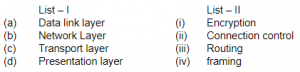A (a)-(iv), (b)-(iii), (c)-(i), (d)-(ii) B (a)-(iii), (b)-(iv), (c)-(ii), (d)-(i) C (a)-(iv), (b)-(ii), (c)-(iii), (d)-(i) D (a)-(iv), (b)-(iii), (c)-(ii), (d)-(i)
Computer-Networks       OSI-TCP-layers
Question 28 Explanation:
Network layer→ Routing
Transport layer→ Connection control
Presentation layer→ Encryption
 Question 29
The address of a class B host is to be split into subnets with a 6 - bit subnet number. What is the maximum number of subnets and maximum number of hosts in each subnet?
 A 62 subnets and 1022 hosts B 64 subnets and 1024 hosts C 62 subnets and 254 hosts D 64 subnets and 256 hosts
Computer-Networks       Subnetting
Question 29 Explanation:
It is a class B address, so there 16-bits for NID and 16-bits for HID.
From HID, we took 6-bits for subnetting.
Then total subnets possible = ( 26 ) - 2 = 64
Total hosts possible for each subnet = (210) - 2 = 1022
 Question 30
Which of the following statements are true?
(a) The fragmentation fields in the base header section of IPv4 have moved to the fragmentation extension header in IPv6.
(b) The authentication extension header is new in IPv6.
(c) The record route option is not implemented in IPv6.
 A (a) and (b) Only B (b) and (c) Only C (a) and (c) Only D (a), (b) and (c)
Question 30 Explanation: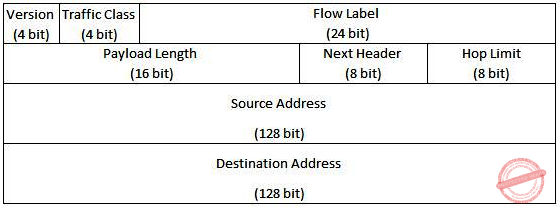Question 31
Consider a full binary tree with n internal nodes, internal path length i, and external path length e. The internal path length of a full binary tree is the sum, taken over all nodes of the tree, of the depth of each node. Similarly, the external path length is the sum, taken over all leaves of the tree, of the depth of each leaf. Which of the following is correct for the full binary tree?
 A e = i+n B e = i+2n C e = 2i+n D e = 2n+i
Data-Structures       Binary-Trees
Question 31 Explanation:
→ A node's path length is the number of links (or branches) required to get back to the root.
→ The root has path length zero and the maximum path length in a tree is called the tree's height.
→ The sum of the path lengths of a tree's internal nodes is called the internal path length and the sum of the path lengths of a tree's external nodes is called the external path length.
External Path Length: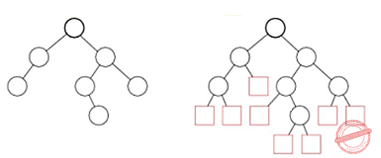The sum over all external (square) nodes of the lengths of the paths from the root of an extended binary tree to each node. For example, in the tree above, the external path length is 25 (Knuth 1997, pp. 399-400). The internal and external path lengths are related by
E = I + 2n,
where n is the number of internal nodes.
 Question 32
You are given a sequence of n elements to sort. The input sequence consists of n/k subsequences,each containing k elements. The elements in a given subsequence are all smaller than the elements in the succeeding subsequence and larger than the elements in the preceding subsequence. Thus, all that is needed to sort the whole sequence of length n is to sort the k elements in each of the n/k subsequences. The lower bound on the number of comparisons needed to solve this variant of the sorting problem is
 A Ω(n) B Ω(n/k) C Ω(nlogk ) D Ω(n/klogn/k)
Algorithms       Sorting
Question 32 Explanation:
There are n/k subsequences and each can be ordered in k! ways. This makes a (k!)n/k outputs. We can use the same reasoning:
(k!)n/k ≤ 2h
Taking the logarithm of both sides, we get:
h ≥ lg(k!)n/k
= (n/k)lg(k!)
≥ (n/k)(k/2)lg(k/2)
= (1/2)*(nlogk)-(1/2)*n
= Ω(nlogk)
 Question 33
T(n)= 8T (n/2)+Cn, if n>1 = b, if n=1 Consider the recurrence relation:   Where b and c are constants. The order of the algorithm corresponding to above recurrence relation is:
 A n B n2 C n log n D n3
Algorithms       Time-Complexity
Question 33 Explanation:
The above recurrence is in the form of masters theorem.
a=8,b=2,k=0 and p=0
Case-1: a>bk = 8>20
T(n)=O(nlogb^a)
= O(n3)
 Question 34
Consider the following two sequences :
X = < B, C, D, C, A, B, C > and
Y = < C, A, D, B, C, B >
The length of longest common subsequence of X and Y is :
 A 5 B 3 C 4 D 2
Algorithms       Dynamic-Programming
Question 34 Explanation: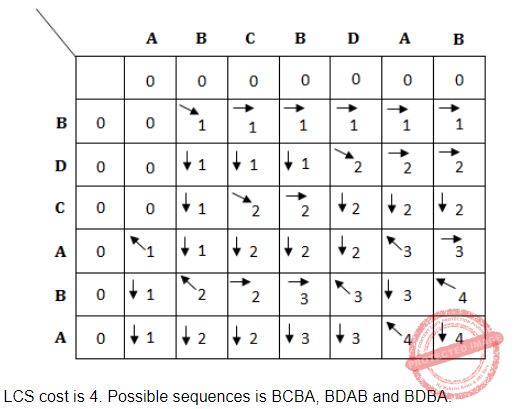Question 35
A text is made up of the characters a, b, c, d, e each occurring with the probability 0.11, 0.40, 0.16, 0.09 and 0.24 respectively. The optimal Huffman coding technique will have the average length of:
 A 2.4 B 2.16 C 2.26 D 2.15
Algorithms       Greedy-approach
Question 35 Explanation: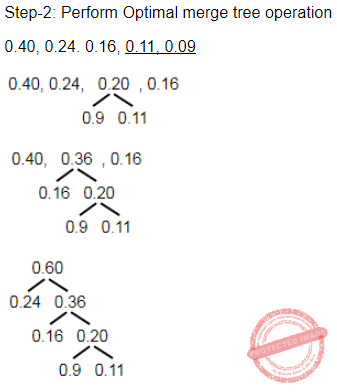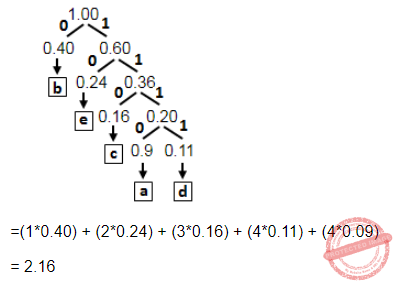Question 36
An undirected graph G (V, E) contains n (n > 2) nodes named v1 , v2 ,...,vn. Two nodes vi and vj are connected if and only if 0 <│i−j│≤2. Each edge (vi , vj ) is assigned a weight i+j. The cost of the minimum spanning tree of such a graph with 10 nodes is:
 A 88 B 91 C 49 D 21
Algorithms       Minimum-Spanning-Tree
Question 36 Explanation:
Method-1: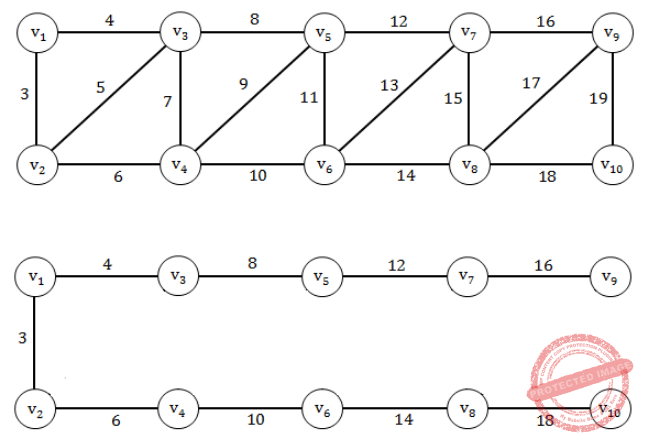Question 37
An XML document that adheres to syntax rules specified by XML 1.0 specification in that it must satisfy both physical and logical structured, is called:
 A Well - formed B Reasonable C Valid D Sophisticated
Web-Technologies       XML
Question 37 Explanation:
A well-formed document in XML is a document that "adheres to the syntax rules specified by the XML 1.0 specification in that it must satisfy both physical and logical structures".
Well formed documents requirements:
1. Content be defined.
2. Content be delimited with a beginning and end tag
3. Content be properly nested (parents within roots, children within parents)
 Question 38
Which of the following statement(s) is/are TRUE regarding Java Servlets ?
(a) A Java Servlet is a server-side component that runs on the web server and extends the capabilities of a server.
(b) A Servlet can use the user interface classes like AWT or Swing.
 A Only (a) is TRUE. B Only (b) is TRUE. C Both (a) and (b) are TRUE. D Neither (a) nor (b) is TRUE.
Web-Technologies       Java-Script
Question 38 Explanation:
TRUE: A Java Servlet is a server-side component that runs on the web server and extends the capabilities of a server.
FALSE: A Servlet can not use the user interface classes like AWT or Swing.
→ Servlets can respond to any types of requests, they most commonly implement web containers for hosting web applications on web servers and thus qualify as a server-side servlet web API.
 Question 39
Consider the following HTML table definition:
<table border = 1>
<tr>
<td> colspan = 2> Text A </td>
</tr>
<tr>
<td> Text B </td>
<td> Text C </td*gt;
</tr>
<tr>
<td rowspan = 2> Text D </td>
</tr>
<tr>
<td> Text E </td>
<tr>
<td> Text F </td>
</tr>
</table>
The above HTML code would render on screen as:
 ABCDWeb-Technologies       HTML
Question 39 Explanation:Question 40
Which of the following statements is/are TRUE ?
(a) In HTML, character entities are used to incorporate external content into a web page, such as images.
(b) Once a web server returns a cookie to a browser, the cookie will be included in all future requests from the browser to the same server.
 A Only (a) is TRUE. B Only (b) is TRUE. C Both (a) and (b) are TRUE. D Neither (a) nor (b) is TRUE.
Web-Technologies       HTML
Question 40 Explanation:
FALSE: Entities are used to display characters that have special HTML meaning, such as “<” and “>”.
FALSE: Once a web server returns a cookie to a browser, the cookie will be included in all future requests from the browser to the same server.
 Question 41
Which of the following statements is/are TRUE regarding JAVA ? (a) Constants that cannot be changed are declared using the ‘static’ keyword. (b) A class can only inherit one class but can implement multiple interfaces.
 A Only (a) is TRUE. B Only (b) is TRUE. C Both (a) and (b) are TRUE. D Neither (a) nor (b) are TRUE.
OOPS       JAVA
Question 41 Explanation:
FALSE: Constants that cannot be changed are declared using the ‘static’ keyword.
TRUE: A class can only inherit one class but can implement multiple interfaces.
 Question 42
What is the output of the following JAVA program ?
Class Test {
public static void main(String[] args) {
Test obj = new Test();
obj.start();
}
void start() {
String stra = ”do”;
String strb = method(stra);
System.out.print(“: ”+stra + strb);
}
String method(String stra) {
stra = stra + ”good”;
System.out.print(stra);
return“ good”;
}
}
 A dogood : dogoodgood B dogood : gooddogood C dogood : dodogood D dogood : dogood
OOPS       Class-and-object
 Question 43
Statistical software quality assurance in software engineering involves __________ .
 A using sampling in place of exhaustive testing of software. B surveying customers to find out their opinions about product quality. C tracing each defect to its underlying cause, isolating the vital few causes, and moving to correct them. D tracing each defect to its underlying causes, and using the Pareto principle to correct each problem found.
Software-Engineering       Software-quality
Question 43 Explanation:
→ Statistical software quality assurance in software engineering involves tracing each defect to its underlying cause, isolating the vital few causes, and moving to correct them.
→ Statistical software quality assurance uses pareto principle to identify vital causes (80% of defects can be traced to 20% of causes) and moves to correct the problems that have caused the defects.
 Question 44
Which of the following statements is/are FALSE with respect to software testing ?
S1 : White-box tests are based on specifications; better at telling whether program meets specification, better at finding errors of omission.
S2 : Black- box tests are based on code; better for finding crashes, out of bounds errors, file not closed errors.
S3 : Alpha testing is conducted at the developer’s site by a team of highly skilled testers for software that is developed as a product to be used by many customers.
 A Only S1 and S2 are FALSE. B Only S1 and S3 are FALSE. C Only S2 and S3 are FALSE. D All of S1, S2, and S3 are FALSE.
Software-Engineering       Software-testing
Question 44 Explanation:
FALSE: Black box tests are based on specifications; better at telling whether program meets specification, better at finding errors of omission.
FALSE: White box tests are based on code; better for finding crashes, out of bounds errors, file not closed errors.
TRUE: Alpha testing is conducted at the developer’s site by a team of highly skilled testers for software that is developed as a product to be used by many customers.
 Question 45
A signal processor software is expected to operate for 91.25 days after repair, and the mean software repair time is expected to be 5 minutes. Then, the availability of the software is:
 A 96.9862% B 97.9862% C 98.9962% D 99.9962%
Software-Engineering       Software-quality
Question 45 Explanation:
Given data,
-- Software expected to operate after repair(MTBF) = 91.25 days
= 91.25 * 24 hours
= 2190 hours
-- Mean software repair time(MTTR) = 5 mins
= 5/60 hrs
= 0.083 hrs
-- Software Availability=?
Step-1: Software Availability =MTBF / (MTBF + MTTR)
= [2190 / (2190 + 0.083)]*100
= (2190 / 2190.083)*100
= 0.9996211481 * 100
= 99.9962%
 Question 46
Consider the method mcq ( ):
int mcq(boolean a, boolean b, boolean c, boolean d)
{
int ans=1;
if(a) { ans = 2; }
else if (b) { ans = 3; }
else if (c) {
if (d) { ans = 4; }
return ans;
}
if
M1 = Number of tests to exhaustively test mcq( );
M2 = Minimum number of tests to achieve full statement coverage for mcq( ); and
M3 = Minimum number of tests to achieve full branch coverage for mcq( );
then (M1, M2, M3) = __________.
 A (16, 3, 5) B (8, 5, 3) C (8, 3, 5) D (16, 4, 4)
Software-Engineering       Software-testing
Question 46 Explanation:Question 47
A simple stand - alone software utility is to be developed in ‘C’ programming by a team of software experts for a computer running Linux and the overall size of this software is estimated to be 20,000 lines of code. Considering (a, b) = (2.4, 1.05) as multiplicative and exponention factor for the basic COCOMO effort estimation equation and (c, d) = (2.5, 0.38) as multiplicative and exponention factor for the basic COCOMO development time estimation equation, approximately how long does the software project take to complete ?
 A 10.52 months B 11.52 months C 12.52 months D 14.52 months
Software-Engineering       COCOMO-Model
Question 47 Explanation:
Given data,
-- lines of code(LOC)= 20,000 (or 20K)
-- Multiplicative value ‘a’=2.4
-- Exponential ‘b’=1.05
-- Basic COCOMO formula= a*(LOC in K)b
-- Multiplicative value ‘c’=2.5
-- Exponential ‘d’=0.38
-- Development=c*(basic COCOMO result)d
Step-1: Basic COCOMO formula= a*(LOC in K)b
= 2.4*(20)1.05
= 55.756
Step-2: Development= c*(basic COCOMO result)d
= 2.5(55.756)0.38
= 11.52 months
 Question 48
In Software Configuration Management (SCM), which of the following is a use-case supported by standard version control systems ?
(a) Managing several versions or releases of a software
(b) Filing bug reports and tracking their progress
(c) Allowing team members to work in parallel
(d) Identifying when and where a regression occurred
 A Only (a), (c), and (d) B Only (a), (b), and (c) C Only (a), (b), and (d) D Only (b), (c), and (d)
Software-Engineering       Software-configuration-management
Question 48 Explanation:
Software Configuration Management (SCM) is a use-case supported by standard version control systems:
1. Develop the next version of a piece of software while fixing problems with the current one.
2. Share code with other team members in a controlled way, allowing you to develop code in parallel with others and join with the current state of the codeline.
3. Identify what versions of code went into a particular component.
4. Analyze where change happened in the history of a component's development.
 Question 49
Consider the following four processes with the arrival time and length of CPU burst given in milliseconds :The average waiting time for preemptive SJF scheduling algorithm is __________.
 A 6.5 B 7.5 C 6.75 D 7.75
Operating-Systems       Process-Scheduling
Question 49 Explanation:Question 50
Consider a virtual page reference string 7, 0, 1, 2, 0, 3, 0, 4, 2, 3, 0, 3, 2, 1, 2, 0, 1, 7, 0, 1 Suppose a demand paged virtual memory system running on a computer system such that the main memory has 3 page frames. Then __________ page replacement algorithm has minimum number of page faults.
 A FIFO B LIFO C LRU D Optimal
Operating-Systems       Page-Replacement-algorithm
Question 50 Explanation:Question 51
User level threads are threads that are visible to the programmer and are unknown to the kernel. The operating system kernel supports and manages kernel level threads. Three different types of models relate user and kernel level threads. Which of the following statements is/are true ?
(a) (i) The Many - to - one model maps many user threads to one kernel thread
(ii) The one - to - one model maps one user thread to one kernel thread
(iii) The many - to - many model maps many user threads to smaller or equal kernel threads
(b) (i) Many - to - one model maps many kernel threads to one user thread
(ii) One - to - one model maps one kernel thread to one user thread
(iii) Many - to - many model maps many kernel threads to smaller or equal user threads
 A (a) is true; (b) is false B (a) is false; (b) is true C Both (a) and (b) are true D Both (a) and (b) are false
Question 51 Explanation:Question 52
Consider a system with five processes P0 through P4 and three resource types A, B and C. Resource type A has seven instances, resource type B has two instances and resource type C has six instances suppose at time T0we have the following allocation.If we implement Deadlock detection algorithm we claim that system is ____________.
(1) Semaphore
(3) Circular wait
 A (1), (2) and (3) B (1) and (3) C (3) and (4) D All are correct.
Operating-Systems       Process-Synchronization
Question 52 Explanation: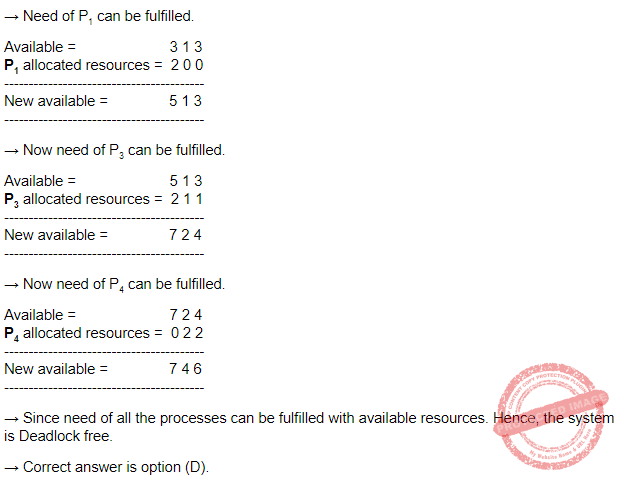Question 53
Consider a disk queue with requests for I/O to blocks on cylinders
98, 183, 37, 122, 14, 124, 65, 67
Suppose SSTF disk scheduling algorithm implemented to meet the requests then the total number of head movements are __________ if the disk head is initially at 53.
 A 224 B 248 C 236 D 240
Operating-Systems       Disk-Scheduling
Question 53 Explanation:Question 54
The Bounded buffer problem is also known as __________.
 A Producer - consumer problem B Reader - writer problem C Dining Philosophers problem D Both (2) and (3)
Operating-Systems       Process-Synchronization
Question 54 Explanation:
→ The Bounded buffer problem is also known as producer-consumer problem.
→ The problem describes two processes, the producer and the consumer, who share a common, fixed-size buffer used as a queue.
→ The producer's job is to generate data, put it into the buffer, and start again. At the same time, the consumer is consuming the data (i.e., removing it from the buffer), one piece at a time.
→ The problem is to make sure that the producer won't try to add data into the buffer if it's full and that the consumer won't try to remove data from an empty buffer.
 Question 55
In Artificial Intelligence (AI), which agent deals with happy and unhappy state?
 A Simple reflex agent B Model based agent C Learning agent D Utility based agent
Question 55 Explanation:
Simple reflex agents
Simple reflex agents act only on the basis of the current percept, ignoring the rest of the percept history. The agent function is based on the condition-action rule: "if condition, then action".
Model-based reflex agents: A model-based agent can handle partially observable environments. Its current state is stored inside the agent maintaining some kind of structure which describes the part of the world which cannot be seen. This knowledge about "how the world works" is called a model of the world, hence the name "model-based agent".
Utility-based agents: Goal-based agents only distinguish between goal states and non-goal states. It is possible to define a measure of how desirable a particular state is. This measure can be obtained through the use of a utility function which maps a state to a measure of the utility of the state. A more general performance measure should allow a comparison of different world states according to exactly how happy they would make the agent. The term utility can be used to describe how "happy" the agent is.
Learning agents: Learning has the advantage that it allows the agents to initially operate in unknown environments and to become more competent than its initial knowledge alone might allow. The most important distinction is between the "learning element", which is responsible for making improvements, and the "performance element", which is responsible for selecting external actions.
 Question 56
If b is the branching factor and m is the maximum depth of the search tree, what is the space complexity of greedy search?
 A O(b+m) B O(bm) C O(bm) D O(mm)
Algorithms       Greedy-Search
Question 56 Explanation:Question 57
Let P, Q, R and S be Propositions. Assume that the equivalences P ⇔ (Q ∨ ¬ Q) and      Q ⇔ R hold. Then the truth value of the formula (P ∧ Q) ⇒ ((P ∧ R) ∨ S) is always:
 A True B False C Same as truth table of Q D Same as truth table of S
Engineering-Mathematics       Propositional-Logic
Question 57 Explanation:
Given data,
-- Equivalence condition(Both RHS and LHS should be TRUE)= P ⇔ (Q V ~Q)
-- Holding condition(Both RHS and LHS may TRUE/FALSE)= Q ⇔ RQuestion 58
“If X, then Y unless Z” is represented by which of the following formulae in propositional logic?
 A (X ∧ Y) → ¬ Z B (X ∧ ¬ Z) → Y C X → (Y ∧ ¬ Z) D Y → (X ∧ ¬ Z)
Engineering-Mathematics       Propositional-Logic
Question 58 Explanation:
"If X then Y unless Z" ⇒ ¬Z → (X→Y)
⇒ Z ∨ ¬X ∨ Y
⇒ ¬X ∨ Z ∨ Y
Option B: (X ∧ ¬Z) → Y
= ¬(X ∧ ¬Z ) ∨ Y
= ¬X ∨ Z ∨ Y
Hence, option (B) is correct.
 Question 59
Consider the following two well-formed formulas in propositional logic.
F1 : P ⇒ ¬ P
F2 : (P ⇒ ¬ P) ∨ (¬ P ⇒ P)
Which of the following statements is correct?
 A F1 is Satisfiable, F2 is valid B F1 is unsatisfiable, F2 is Satisfiable C F1 is unsatisfiable, F2 is valid D F1 and F2 both are Satisfiable
Engineering-Mathematics       Propositional-Logic
Question 59 Explanation:Question 60
Standard planning algorithms assume environment to be __________.
 A Both deterministic and fully observable B Neither deterministic nor fully observable C Deterministic but not fully observable D Not deterministic but fully observable
Artificial-intelligence       Planning
Question 60 Explanation:
→ Classical planning environments that are fully observable, deterministic, finite, static and discrete (in time, action, objects and effects).
 Question 61
Which of the following statements is not correct?
 A Every recursive language is recursively enumerable. B L = {0n1n 0n │n=1, 2 , 3, ....} is recursively enumerable. C Recursive languages are closed under intersection. D Recursive languages are not closed under intersection.
Theory-of-Computation       Recursive-and-Recursively-Enumerable-Language
Question 61 Explanation:
TRUE: Every recursive language is recursively enumerable.
TRUE: L = {0n1n 0n │n=1, 2 , 3, ....} is recursively enumerable.
TRUE: Recursive languages are closed under intersection.
→ Recursive closed under union,intersection,complementation,etc.., except Homomorphism and substitution.
FALSE: Recursive languages are not closed under intersection.
→ Recursively enumerable languages are closed under union,intersection,etc.., except set difference and complementation.
 Question 62
Context free grammar is not closed under:
 A Concatenation B Complementation C Kleene Star D Union
Theory-of-Computation       Context-Free-Grammar
Question 62 Explanation:
→ Context-free grammar are closed under Union,Concatenation and Kleene closure.
→ Context-free grammar are not closed under set difference,Complementation and intersection.
 Question 63
Consider the following languages: L1 = {am bn │ m ≠ n} L2 = {am bn │ m = 2n+1} L3 = {am bm │ m ≠ 2n} Which one of the following statement is correct ?
 A Only L1 and L2 are context free languages B Only L1 and L3 are context free languages C Only L2 and L3 are context free languages D L1, L2 and L3 are context free languages
Theory-of-Computation       Context-Free-Language
Question 63 Explanation:
L1={am bn | m≠n }
→ It means m could be greater than or less than ‘n’ but m will not be equal to ‘n’.
→ Since for accepting the language L1 we need a storage so then no. of a’s can be stored in it and when b’s come as input a’s and b’s can be compared.
→ Since finite automata do not have a storage element hence the language is not regular.
→ Now state transition diagram to check whether L1 is CFL or not:→ Hence L2 is also a CFL.
L3: First simply read one 'a', then push one 'a' in the stack after reading two a's and then pop all the a's by reading the b's. Since can be done by PDA hence CFL.
 Question 64
A 4*4 DFT matrix is given by :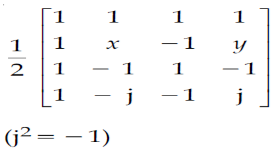Where values of x and y are _____, _____ respectively.
 A 1, −1 B −1, 1 C −j, j D j, −j
Computer-Graphics       DFT-Matrix
 Question 65
Entropy of a discrete random variable with possible values {x1, x2, ..., xn} and probability density function P(X) is :The value of b gives the units of entropy. The unit for b=10 is :
 A bits B bann C nats D deca
Artificial-intelligence       Entropy
 Question 66
For any binary (n, h) linear code with minimum distance (2t+1) or greaterA 2t+1 B t+1 C t D t-1
Artificial-intelligence       Linear-Distance
 Question 67
Which of the following is a valid reason for causing degeneracy in a transportation problem ?
Here m is no. of rows and n is no. of columns in transportation table
 A When the number of allocations is m+n−1. B When two or more occupied cells become unoccupied simultaneously. C When the number of allocations is less than m+n−1. D When a loop cannot be drawn without using unoccupied cells, except the starting cell of the loop.
LPP       Transportation-Problem
 Question 68
Consider the following LPP :
Max Z = 15x1 + 10x2
Subject to the constraints
4x1 + 6x2 ≤ 360
3x1 + 0x2 ≤ 180
0x1 + 5x2 ≤ 200
x1, x2 / 0
The solution of the LPP using Graphical solution technique is :
 A x1=60, x2=0 and Z=900 B x1=60, x2=20 and Z=1100 C x1=60, x2=30 and Z=1200 D x1=50, x2=40 and Z=1150
LPP       Graphical-Solution-Technique
 Question 69
Consider the following LPP :
Min Z = 2x1 + x2 + 3x3
Subject to :
x1 − 2x2 + x3 ≥ 4
2x1 + x2 + x3 ≤ 8
x1 − x3 ≥ 0
x1, x2, x3 ≥ 0
The solution of this LPP using Dual Simplex Method is :
 A x1=0, x2=0, x3=3 and Z=9 B x1=0, x2=6, x2=0 and Z=6 C x1=4, x2=0, x2=0 and Z=8 D x1=2, x2=0, x2=2 and Z=10
LPP       Dual-Simplex-Method
 Question 70
Consider a Takagi - Sugeno - Kang (TSK) Model consisting rules of the form: If Xi is Ai1 and... and xr is Air THEN y = fi(x1, x2,.... xr) = bi0 + bi1x1 + birxr assume, ai is the matching degree of rule i, then the total output of the model is given by:
 ABCD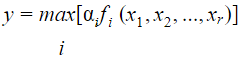Artificial-intelligence       Takagi-Sugeno-Kang (TSK)-Model
 Question 71
Consider a single perceptron with sign activation function. The perceptron is represented by weight vector [0.4 −0.3 0.1]t and a bias θ = 0. If the input vector to the perceptron is X = [0.2 0.6 0.5] then the output of the perceptron is:
 A 1 B 0 C -0.25 D -1
Artificial-intelligence       Perceptron
 Question 72
The Sigmoid activation function f(t) is defined as:
 ABCDArtificial_Intelligence       Sigmoid_function
 Question 73
Consider the following statements :
(a) UNIX provides three types of permissions
* Write
* Execute
(b) UNIX provides three sets of permissions
* permission for owner
* permission for group
* permission for others
Which of the above statement/s is/are true ?
 A only (a) B only (b) C Both (a) and (b) D Neither (a) nor (b)
Operating-Systems       UNIX-Operating-System
Question 73 Explanation:
→ UNIX provides three types of permissions using octal numbers
2. Write(W or 2)
3. Execute(X or 1)
→ UNIX provides three sets of permissions. Each set of permission we are having 3 types of permissions.
1. Permission for owner(RWX or 421)
2. Permission for group(RWX or 421)
3. Permission for others(RWX or 421)
→ We can change/modify permissions using chmod, chown,etc.., commands
→ We can see the permissions for every file using ls -l command
 Question 74
Which of the following routing technique / techniques is / are used in distributed systems ?
(a) Fixed Routing
(b) Virtual Routing
(c) Dynamic Routing
 A (a) only B (a) and (b) only C (c) only D All (a), (b), (C)
Computer-Networks       Routing
Question 74 Explanation:
Distributed systems are using fixed routing only.
Fixed routing: A path from A to B is specified in advance; path changes only if a hardware failure disables it.
1. Since the shortest path is usually chosen, communication costs are minimized.
3. Ensures that messages will be delivered in the order in which they were sent
Virtual routing: A path from A to B is fixed for the duration of one session.
1. Different sessions involving messages from A to B may have different paths
3. Ensures that messages will be delivered in the order in which they were sent
Dynamic routing: The path used to send a message form site A to site B is chosen only when a message is sent
1. Usually a site sends a message to another site on the link least used at that particular time
2. Adapts to load changes by avoiding routing messages on heavily used path
3. Messages may arrive out of order; This problem can be remedied by appending a sequence number to each message
4. Most complex to set up
 Question 75
Match the following WINDOWS system calls and UNIX system calls with reference to process control and File manipulation.A (a)-(iii), (b)-(iv), (c)-(i), (d)-(ii) B (a)-(iv), (b)-(iii), (c)-(i), (d)-(ii) C (a)-(iv), (b)-(iii), (c)-(ii), (d)-(i) D (a)-(iii), (b)-(iv), (c)-(ii), (d)-(i)
Operating-Systems       UNIX-Operating-System
Question 75 Explanation:
In UNIX Wait() system call is equivalent to windows system call is WaitForSingleObject() .
In UNIX Fork() system call is equivalent to windows system call is Create-process().
In UNIX Create() system call is equivalent to windows system call is CreateFile().
In UNIX Close() system call is equivalent to windows system call is CloseHandle()
There are 75 questions to complete.